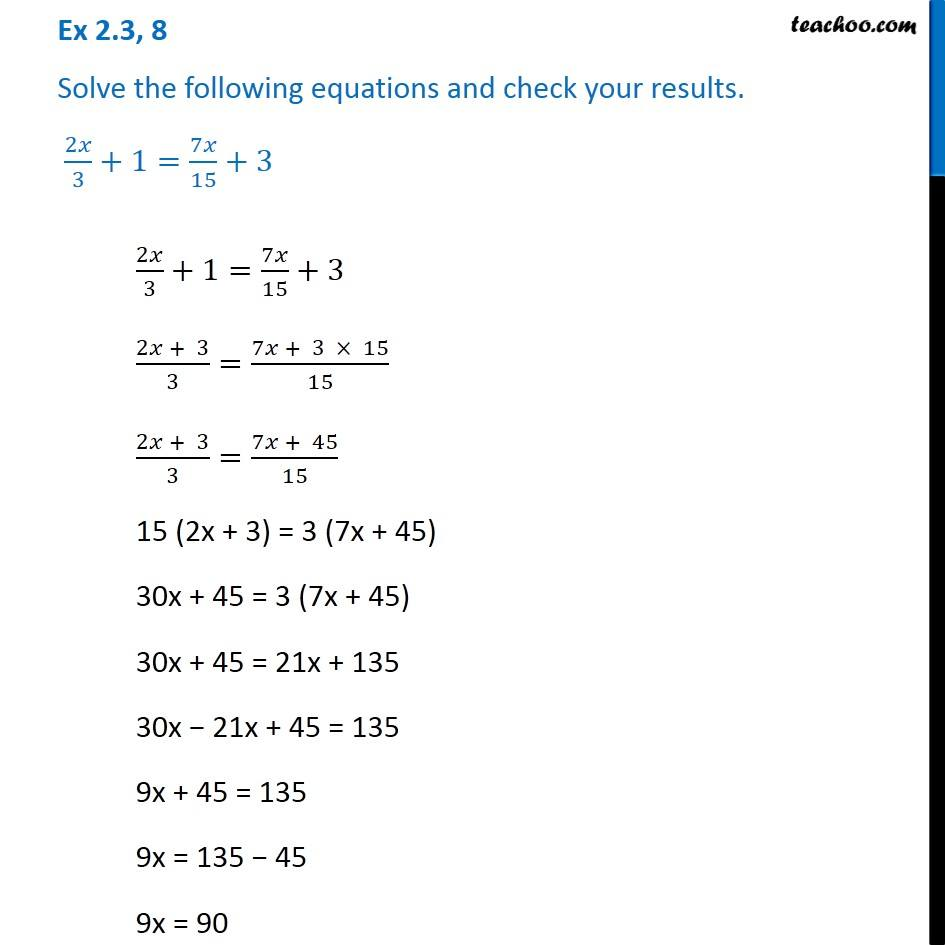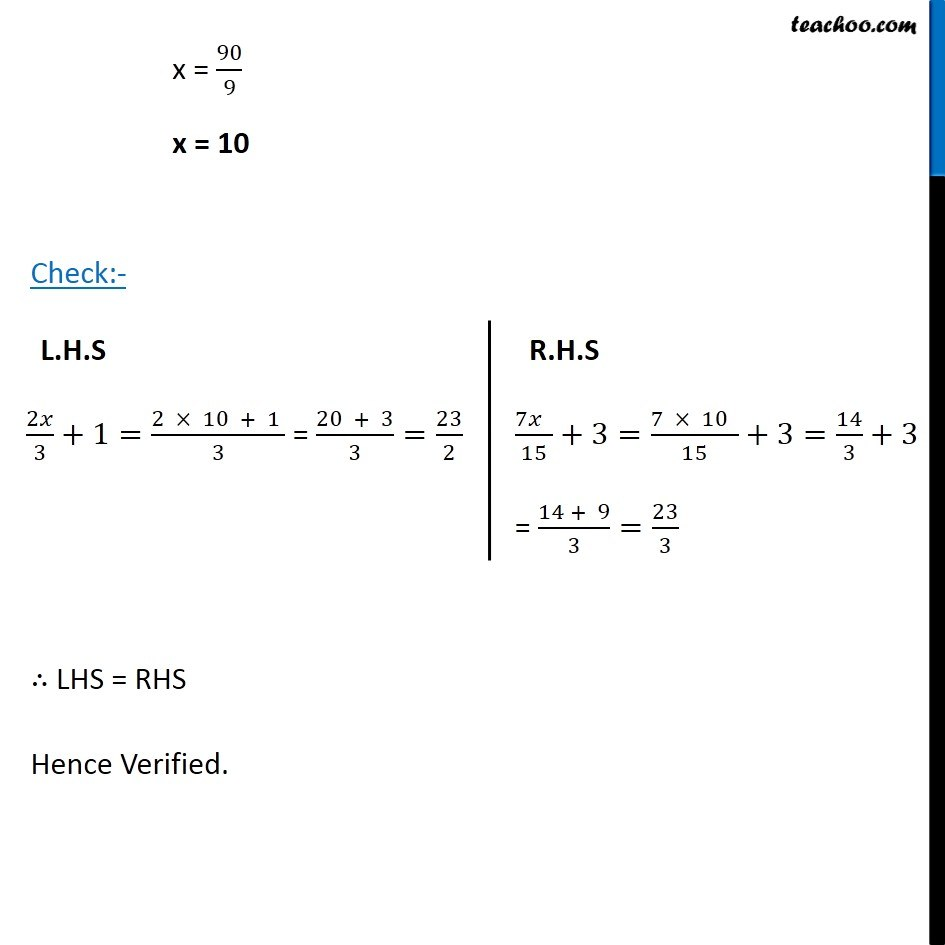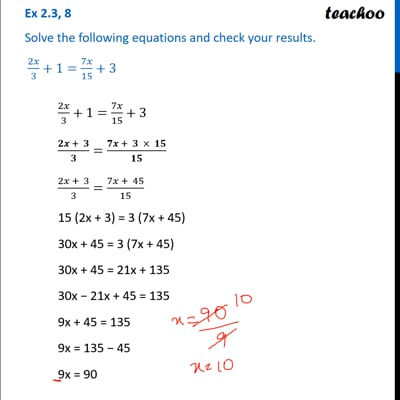Ex 2.3

Chapter 2 Class 8 Linear Equations in One Variable
Serial order wiseThis video is only available for Teachoo black users

Get live Maths 1-on-1 Classs - Class 6 to 12

### Transcript

Ex 2.3, 8 Solve the following equations and check your results. 2𝑥/3+1=7𝑥/15+3 2𝑥/3+1=7𝑥/15+3 (2𝑥 + 3)/3=(7𝑥 + 3 × 15)/15 (2𝑥 + 3)/3=(7𝑥 + 45)/15 15 (2x + 3) = 3 (7x + 45) 30x + 45 = 3 (7x + 45) 30x + 45 = 21x + 135 30x − 21x + 45 = 135 9x + 45 = 135 9x = 135 − 45 9x = 90 x = 90/9 x = 10 Check:- ∴ LHS = RHS Hence Verified. L.H.S 2𝑥/3+1=(2 × 10 + 1 )/3 = (20 + 3)/3=23/2 R.H.S (7𝑥 )/15+3=(7 × 10 )/15+3=14/3+3 = (14 + 9)/3=23/3